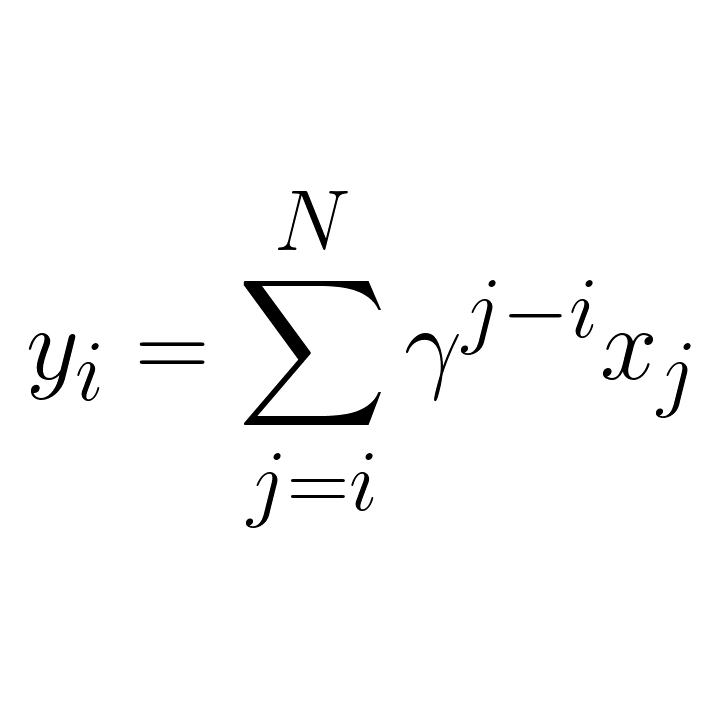Fast Discounted Cumulative Sums in PyTorch

torch-discounted-cumsum

Overview

This landing page provides a brief overview of an efficient parallel algorithm for the computation of discounted cumulative sums and a Python package with differentiable bindings to PyTorch. The discounted `cumsum` operation is frequently seen in data science domains concerned with time series, including Reinforcement Learning (RL). For implementation details and other characteristics, please visit the project GitHub .

The traditional sequential algorithm performs the computation of the output elements in a loop. For an input of size `N`, it requires `O(N)` operations and takes `O(N)` time steps to complete.

The proposed parallel algorithm requires a total of `O(N log N)` operations, but takes only `O(log N)` time steps, which is a considerable trade-off in many applications involving large inputs.

Features of the parallel algorithm:

• Speed logarithmic in the input size
• Better numerical precision than sequential algorithms

Features of the package:

• CPU: sequential algorithm in C++
• GPU: parallel algorithm in CUDA
• Both left and right directions of summation supported
• PyTorch bindings

Parallel Algorithm

For the sake of simplicity, the algorithm is explained for `N=16`. The processing is performed in-place in the input vector in `log2 N` stages. Each stage updates `N / 2` positions in parallel (that is, in a single time step, provided unrestricted parallelism). A stage is characterized by the size of the group of sequential elements being updated, which is computed as `2 ^ (stage - 1)`. The group stride is always twice larger than the group size. The elements updated during the stage are highlighted with the respective stage color in the figure below. Here input elements are denoted with their position id in hex, and the elements tagged with two symbols indicate the range over which the discounted partial sum is computed upon stage completion.

Each element update includes an in-place addition of a discounted element, which follows the last updated element in the group. The discount factor is computed as gamma raised to the power of the distance between the updated and the discounted elements. In the figure below, this operation is denoted with tilted arrows with a greek gamma tag. After the last stage completes, the output is written in place of the input.In the CUDA implementation, `N / 2` CUDA threads are allocated during each stage to update the respective elements. The strict separation of updates into stages via separate kernel invocations guarantees stage-level synchronization and global consistency of updates.

Source code

Check out the project GitHub page

```pip install torch-discounted-cumsum
```@misc{obukhov2021torchdiscountedcumsum,